Open in App
Not now

# Class 8 RD Sharma Solutions- Chapter 16 Understanding Shapes Quadrilaterals – Exercise 16.1 | Set 2

• Last Updated : 08 Apr, 2021

### Question 13. In Figure, find the measure of ∠MPN.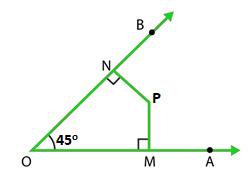Solution:

As we know that Sum of angles of a quadrilateral is = 360°

∠NOP = 45°, ∠OMP = ∠PNO = 90° (Given)

Let us assume that angle ∠MPN is x°

∠NOP + ∠OMP + ∠PNO + ∠MPN = 360°

45° + 90° + 90° + x° = 360°

x° = 360° – 225°

x° = 135°

Hence, Measure of ∠MPN is 135°

### Question 14. The sides of a quadrilateral are produced in order. What is the sum of the four exterior angles?

Solution: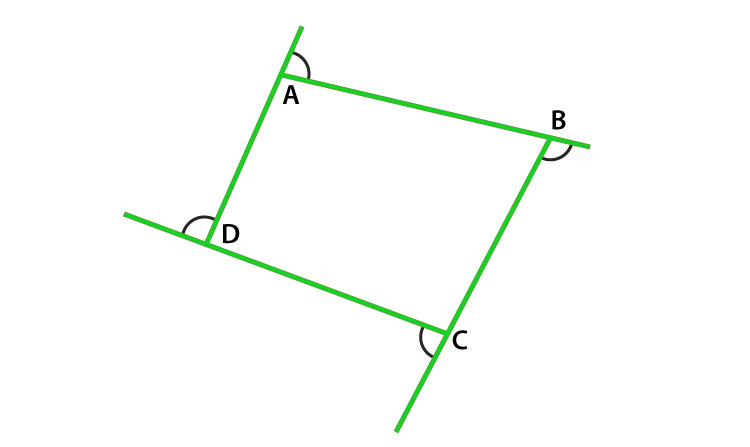As we know that, exterior angle + interior adjacent angle = 180° (Linear pair)

Applying relation for polygon having n sides

Sum of all exterior angles + Sum of all interior angles = n × 180°

Sum of all exterior angles = n × 180° – Sum of all interior angles

= n × 180° – (n -2) × 180° (Sum of interior angles is = (n – 2) x 180°)

= n × 180° – n × 180° + 2 × 180°

= 180°n – 180°n + 360° = 360°

Hence, Sum of four exterior angles is 360o

### Question 15. In Figure, the bisectors of ∠A and ∠B meet at a point P. If ∠C =100° and ∠D = 50°, find the measure of ∠APB.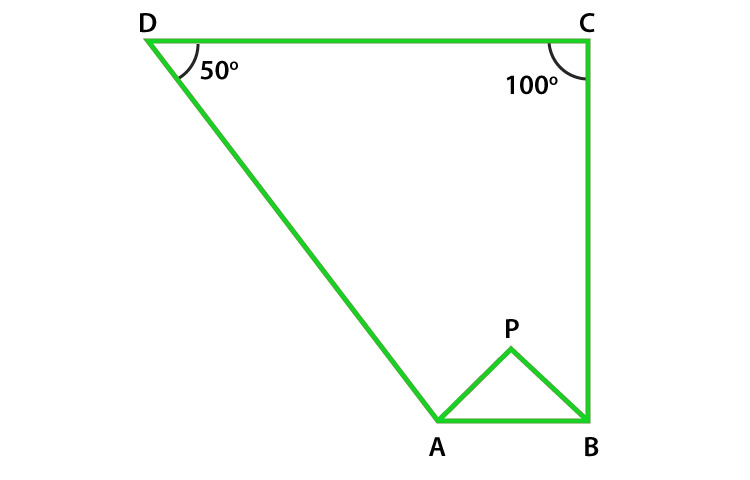Solution:

As we know that Sum of angles of a quadrilateral is = 360°

Given that,

∠C =100° and ∠D = 50°

∠A + ∠B + ∠C + ∠D = 360o

∠A + ∠B + 100o + 50o = 360o

∠A + ∠B = 360o – 150o

∠A + ∠B = 210o (Equation 1)

Now in Δ APB

½ ∠A + ½ ∠B + ∠APB = 180o (sum of triangle is 180o)

∠APB = 180o – ½ (∠A + ∠B) (Equation 2)

On substituting value of ∠A + ∠B = 210 from equation (1) in equation (2)

∠APB = 180o – ½ (210o)

= 180o – 105o = 75o

Hence, the measure of ∠APB is 75o.

### Question 16. In a quadrilateral ABCD, the angles A, B, C and D are in the ratio 1:2:4:5. Find the measure of each angle of the quadrilateral.

Solution:

As we know that Sum of angles of a quadrilateral is = 360°

Let each angle be xo

Therefore,

xo + 2xo + 4xo + 5xo = 360o

12xo = 360o

xo = 360o/12 = 30o

Value of angles are as x = 30o,

2x = 2 × 30 = 60o

4x = 4 × 30 = 120o

5x = 5 × 30 = 150o

Hence, Value of angles are 30o, 60o, 120o, 150o.

### Question 17. In a quadrilateral ABCD, CO and DO are the bisectors of ∠C and ∠D respectively. Prove that ∠COD = 1/2 (∠A +∠B).

Solution:

As we know that sum of angles of a quadrilateral is 360°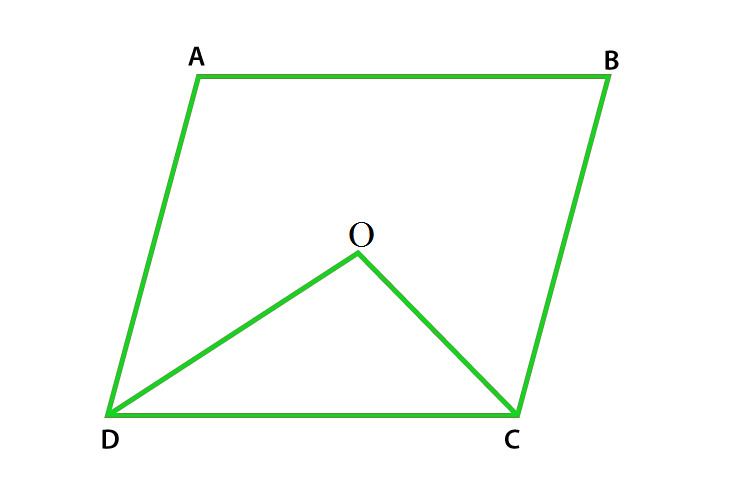Therefore,

∠A + ∠B + ∠C + ∠D = 360o

∠A + ∠B = 360o – (∠C + ∠D)

½ (∠A + ∠B) = ½ [360o – (∠C + ∠D)]

= 180o – ½ (∠C + ∠D) (Equation 1)

Now in Δ DOC

½ ∠D + ½ ∠C + ∠COD = 180o (We know that sum of triangle = 180o)

½ (∠C + ∠D) + ∠COD = 180o

∠COD = 180o – ½ (∠C + ∠D) (Equation 2)

In equations (1) and (2) RHS is equal then LHS will also equal.

Hence, ∠COD = ½ (∠A + ∠B) is proved.

### Question 18. Find the number of sides of a regular polygon, when each of its angles has a measure of

(i) 160°

(ii) 135°

(iii) 175°

(iv) 162°

(v) 150°

Solution:

The sum of interior angle A of a polygon of n sides is given by A = [(n-2) ×180o] /n

(i) 160o

Angle of quadrilateral is 160° (Given)

160o = [(n-2) ×180o]/n

160on = (n-2) ×180o

160on = 180on – 360o

180on – 160on = 360o

20on = 360o

n = 360o/20 = 18

Hence Number of sides are 18

(ii) 135o

Angle of quadrilateral is 135° (Given)

135o = [(n-2) ×180o]/n

135on = (n-2) ×180o

135on = 180on – 360o

180on – 135on = 360o

45on = 360o

n = 360o / 45 = 8

Hence Number of sides are 8

(iii) 175o

Angle of quadrilateral is 175° (Given)

175o = [(n-2) ×180o]/n

175on = (n-2) ×180o

175on = 180on – 360o

180on – 175on = 360o

5on = 360o

n = 360o/5 = 72

Hence Number of sides are 72

(iv) 162o

Angle of quadrilateral is 162° (Given)

162o = [(n-2) ×180o]/n

162on = (n-2) ×180o

162on = 180on – 360o

180on – 162on = 360o

18on = 360o

n = 360o/18 = 20

Hence Number of sides are 20

(v) 150o

Angle of quadrilateral is 160° (Given)

150o = [(n-2) ×180o]/n

150on = (n-2) ×180o

150on = 180on – 360o

180on – 150on = 360o

30on = 360o

n = 360o/30 = 12

Hence Number of sides are 12

### Question 19. Find the numbers of degrees in each exterior angle of a regular pentagon.

Solution:

As we know that the sum of exterior angles of a polygon is 360°

Sum of each exterior angle of a polygon = 360o/n (n is the number of sides)

As we know that number of sides in a pentagon is 5

Sum of each exterior angle of a pentagon = 360o/5 = 72o

Hence Measure of each exterior angle of a pentagon is 72o

### Question 20. The measure of angles of a hexagon are x°, (x-5)°, (x-5)°, (2x-5)°, (2x-5)°, (2x+20)°. Find value of x.

Solution:

As we know that the sum of interior angles of a polygon = (n – 2) × 180° (n = number of sides of polygon)

As we know that hexagon has 6 sides therefore,

The sum of interior angles of a hexagon = (6 – 2) × 180° = 4 × 180° = 720°

x°+ (x-5)°+ (x-5)°+ (2x-5)°+ (2x-5)°+ (2x+20)° = 720°

x°+ x°- 5°+ x° – 5°+ 2x° – 5°+ 2x° – 5°+ 2x° + 20° = 720°

9x° = 720°

x = 720o/9 = 80o

Hence Value of x is 80o

### Question 21. In a convex hexagon, prove that the sum of all interior angle is equal to twice the sum of its exterior angles formed by producing the sides in the same order.

Solution:

As we know that the sum of interior angles of a polygon = (n – 2) × 180°

The sum of interior angles of a hexagon = (6 – 2) × 180° = 4 × 180° = 720°

Sum of exterior angle of a polygon is 360°

Hence Sum of interior angles of a hexagon = Twice the sum of interior angles.

Hence proved.

### Question 22. The sum of the interior angles of a polygon is three times the sum of its exterior angles. Determine the number of sides of the polygon.

Solution:

As we know that the sum of interior angles of a polygon = (n – 2) × 180° (i)

The Sum of exterior angle of a polygon is 360°

therefore,

Sum of Interior Angles = 3 × sum of exterior angles

= 3 × 360° = 1080° (ii)

Now by equating (i) and (ii) we get,

(n – 2) × 180° = 1080°

n – 2 = 1080o/180o

n – 2 = 6

n = 6 + 2 = 8

Hence Number of sides of a polygon is 8.

### Question 23. Determine the number of sides of a polygon whose exterior and interior angles are in the ratio 1 : 5.

Solution:

As we know that the sum of interior angles of a polygon = (n – 2) × 180° (i)

The Sum of exterior angle of a polygon is 360°

As we know that Sum of exterior angles / Sum of interior angles = 1/5 (ii)

By equating (i) and (ii) we get,

360o/(n – 2) × 180° = 1/5

(n – 2) × 180° = 360o × 5

(n – 2) × 180° = 1800o

(n – 2) = 1800o/180o

(n – 2) = 10

n = 10 + 2 = 12

Hence Numbers of sides of a polygon is 12.

### Question 24. PQRSTU is a regular hexagon, determine each angle of ΔPQT.

Solution:

As we know that the sum of interior angles of a polygon = (n – 2) × 180°

The sum of interior angles of a hexagon = (6 – 2) × 180° = 4 × 180° = 720°

Sum of each angle of hexagon = 720o/6 = 120o

∠PUT = 120o Proved.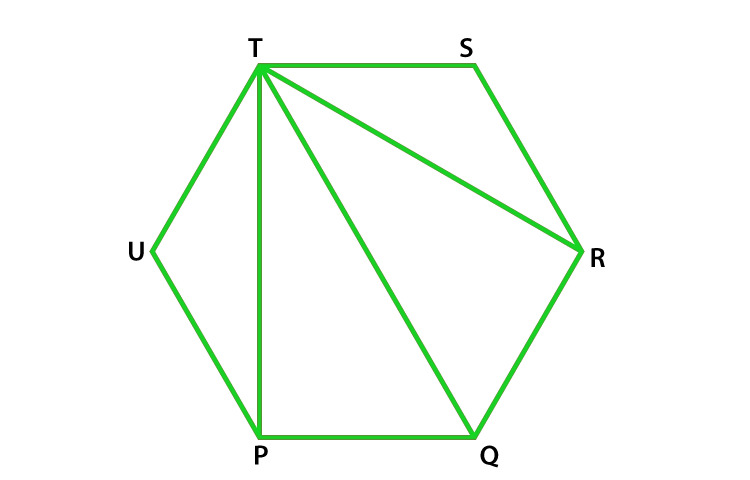In Δ PUT

∠PUT + ∠UTP + ∠TPU = 180o (sum of triangles)

120o + 2∠UTP = 180o (Since Δ PUT is an isosceles triangle )

2∠UTP = 180o – 120o

2∠UTP = 60o

∠UTP = 60o/2 = 30o

∠UTP = ∠TPU = 30o similarly ∠RTS = 30o

therefore ∠PTR = ∠UTS – ∠UTP – ∠RTS

= 120o – 30o – 30o = 60o

∠TPQ = ∠UPQ – ∠UPT

= 120o – 30o = 90o

∠TQP = 180o – 150o = 30o (By using angle sum property of triangle in ΔPQT)

Hence ∠P = 90o, ∠Q = 60o, ∠T = 30o

My Personal Notes arrow_drop_up